Place Value: Ones, Tens & Hundreds Video for Kids | K, 1st & 2nd Grade
1%
It was processed successfully!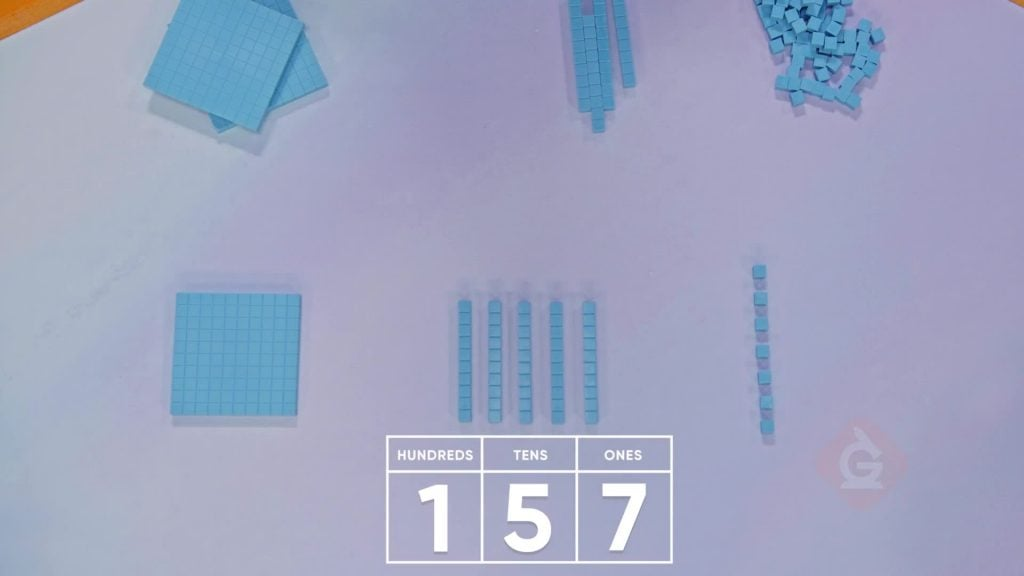WHAT IS PLACE VALUE ONES, TENS & HUNDREDS?

1 ten is the same as 10 ones. 1 hundred is the same as 10 tens. In the number 324, the 3 is in the hundreds place. Its value is 300.

To better understand place value: ones, tens & hundreds…

WHAT IS PLACE VALUE ONES, TENS & HUNDREDS?. 1 ten is the same as 10 ones. 1 hundred is the same as 10 tens. In the number 324, the 3 is in the hundreds place. Its value is 300. To better understand place value: ones, tens & hundreds…

## LET’S BREAK IT DOWN!

### Fruit Punch: Strawberries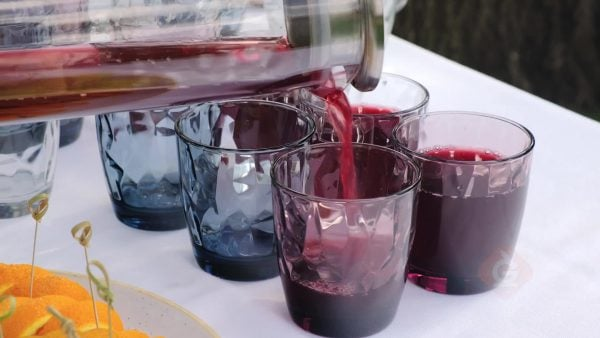99 has 9 tens and 9 ones. Add 1 more one. 9 tens and 10 ones is the same as 10 tens. 10 tens is 1 hundred. 103 has 1 hundred, 0 tens, and 3 ones. Try this one yourself. You have 1 hundreds block and 2 ones blocks. How many blocks do you have? How many strawberries do you have?

Fruit Punch: Strawberries 99 has 9 tens and 9 ones. Add 1 more one. 9 tens and 10 ones is the same as 10 tens. 10 tens is 1 hundred. 103 has 1 hundred, 0 tens, and 3 ones. Try this one yourself. You have 1 hundreds block and 2 ones blocks. How many blocks do you have? How many strawberries do you have?

### Fruit Punch: Blueberries and Raspberries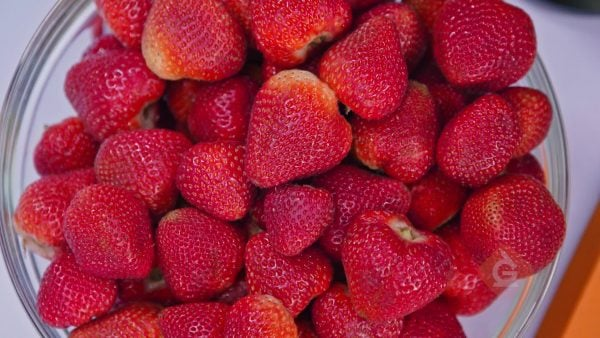You need 123 blueberries for fruit punch. 123 is 1 hundred, 2 tens, and 3 ones. You need 157 raspberries for fruit punch. 157 is 1 hundred, 5 tens, and 7 ones. Try this one yourself. How do you show 143 blackberries with base-ten blocks?

Fruit Punch: Blueberries and Raspberries You need 123 blueberries for fruit punch. 123 is 1 hundred, 2 tens, and 3 ones. You need 157 raspberries for fruit punch. 157 is 1 hundred, 5 tens, and 7 ones. Try this one yourself. How do you show 143 blackberries with base-ten blocks?

### Ball Pit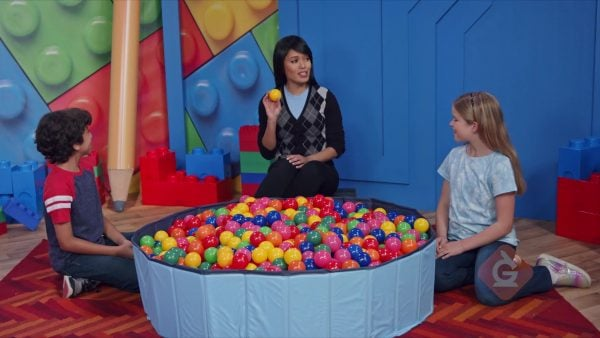There are 359 balls in a ball pit. 359 is 3 hundreds, 5 tens, and 9 ones. Add 1 more ball. Now there are 360 balls in the ball pit. Another pit has 599 balls. Add 1 more. Now there are 600 balls in the ball pit. Try this one yourself. Show 406 with base-ten blocks.

Ball Pit There are 359 balls in a ball pit. 359 is 3 hundreds, 5 tens, and 9 ones. Add 1 more ball. Now there are 360 balls in the ball pit. Another pit has 599 balls. Add 1 more. Now there are 600 balls in the ball pit. Try this one yourself. Show 406 with base-ten blocks.

### Cupcakes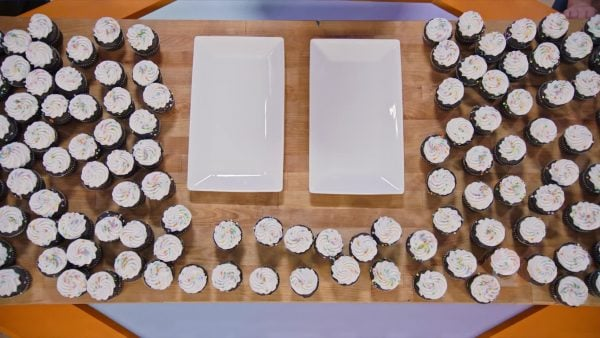You have 230 cupcakes. The 2 is in the hundreds place. You can make 2 groups of 100 cupcakes. The 3 is in the tens place. You can make 3 groups of ten cupcakes. Try this one yourself. How many trays that hold 100 and how many trays that hold 10 do you need for 540 cupcakes?

Cupcakes You have 230 cupcakes. The 2 is in the hundreds place. You can make 2 groups of 100 cupcakes. The 3 is in the tens place. You can make 3 groups of ten cupcakes. Try this one yourself. How many trays that hold 100 and how many trays that hold 10 do you need for 540 cupcakes?

## PLACE VALUE: ONES, TENS & HUNDREDS VOCABULARY

Place value
The value of a digit is based on its position in a number.
Ones
A place in the place-value system that has a value of 1.
Tens
A place in the place-value system that has a value of 10.
Hundreds
A place in the place-value system that has a value of 100.
Digit
A numeral from 0 to 9 that forms part of a number.
More
A greater number has more. When you have 10 more than a number, you are adding 10 to that number.
A lesser number has fewer. When you have 10 fewer than a number, you are subtracting 10 from that number.

## PLACE VALUE: ONES, TENS & HUNDREDS DISCUSSION QUESTIONS

### What is the value of each digit in 378? How do you know?

The digit 3 has a value of 3 hundreds, or 300, because it is in the hundreds place. The digit 7 has a value of 7 tens, or 70, because it is in the tens place. The digit 8 has a value of 8 ones, or 8, because it is in the ones place.

### Represent 256 with base-ten blocks.

2 hundreds blocks, 5 tens blocks, 6 ones blocks.

405.

### Show 3 hundreds, 12 tens, and 5 ones blocks. Ask students to write the number that is represented by the blocks. Have them explain how they got the answer.

425; there are 12 tens. I can’t write a 2-digit number like 12 in the tens place, so I write 2 in the tens place and regroup 10 tens to be 1 hundred. Now I have 1 more hundred in the hundreds place.

### What number do you get when you add 1 to 399? Explain.

When I add 1 to 399, I add 1 to the 9 in the ones place and get 10. I write a 0 in the ones column and regroup 10 ones as 1 ten. Now I have 10 tens, so I write a 0 in the tens column and regroup 10 tens as 1 hundred. Now I have 4 hundreds. So when I add 1 to 399, I get 400.
X

## Success

We’ve sent you an email with instructions how to reset your password.
Ok